# SAT Math Multiple Choice Question 135: Answer and Explanation

### Test Information

Question: 135

15. 17s + 20t = 59
30s + 40t = 110

In the system of equations above, what is the value of t in terms of s ?

• A.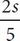• B.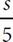• C.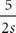• D.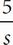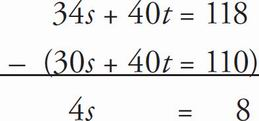So, 4s = 8 and therefore s = 2. Now plug in 2 for the value of s in one of the equations: 30(2) + 40t = 110, so 40t = 110 - 60, so 40t = 50 and t =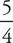= 1.25. Next, plug s = 2 into the answer choices to determine which one matches your target of 1.25: (C),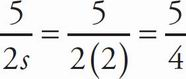= 1.25.